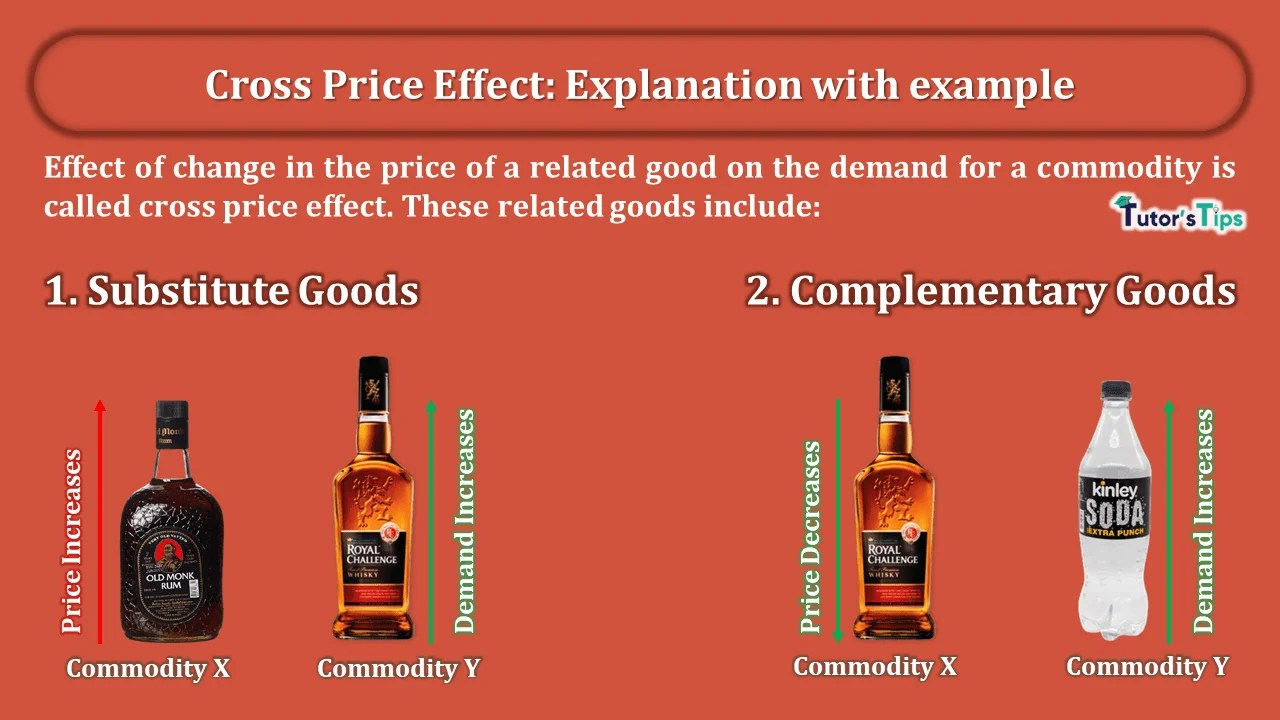# Cross Price Effect: Explanation with exampleCross Price Effect shows the effect of change in the price of commodity-1 on demand for commodity-2 when both the commodities are related goods.

## What is Cross Price Effect:

The effect of change in the price of a related good on the demand for a commodity is called the cross-price effect. These related goods include:

1. Substitute Goods
2. Complementary Goods

Accordingly, we will discuss the effect of both goods differently:

## Demand in relation to the price of Substitute goods:

Substitute goods are those which can be used in place of each other. For example, tea and coffee, Limca and coke, ballpen, and ink pen. As we have discussed in determinants of demand, the increase in the price of one causes the increase in demand of the other and vice versa.

### Illustration showing Cross Price Effect:

We can discuss this with an illustration:

Suppose, Rum and Whisky are two substitute goods. Let whisky be the commodity for which the demand would be affected by the change in the price of Rum.

#### (a) Increase in the price of Rum:

At the initial constant price of Whisky, If the price of Rum in the market increases, the demand for Whisky will go up.

Suppose, the initial price of Whisky is Rs.1,000 and the quantity demanded in the market is 100 units. If the price of Rum in the market increases, the demand for Whisky will increase to 200 units in the market at a constant price of whisky. It is, because the consumers may decide to substitute whisky for rum. Thus, they would buy more whisky. Therefore, the greater purchase of commodity at a constant price point results in a situation of increase in demand, or forward shift in the demand curve. That is why the demand curve shifts to the right, from D1 to D2.

#### (b) Decrease in the price of Rum:

At the initial constant price of Whisky, If the price of Rum in the market decreases, the demand for Whisky will go down.

Suppose, the initial price of Whisky is Rs.1,000 and the quantity demanded in the market is 200 units. If the price of Rum in the market decreases, the demand for Whisky will decrease to 100 units in the market at a constant price of whisky. It is, because the consumers may decide to substitute rum for whisky. Thus, they would buy less whisky. Therefore, the decline in the purchase of commodity at a constant price point to a situation of decrease in demand, or backward shift in the demand curve. That is why the demand curve shifts to the left, from D1 to D2

## Demand in relation to the price of Complementary goods:

Complementary goods are those which are used together to satisfy a want. As we have discussed in determinants of demand, the increase in the price of one causes the decrease in demand of the other and vice versa.

### Illustration showing Cross Price Effect:

We can discuss this with an illustration:

Suppose, Soda and Whisky are two complementary goods. Let soda be the commodity for which the demand would be affected by the change in the price of whisky.

#### (a) Increase in the price of whisky:

At the initial constant price of soda, If the price of whisky in the market increases, the demand for soda will go down.

Suppose, the initial price of Soda is Rs.100 and the quantity demanded in the market is 20 units. If the price of whisky increases, the demand for soda will decrease to 10 units in the market at a constant price of soda. It is, because the consumers may decide to buy less of whisky. Thus, the demand for soda would go down. Therefore, the less purchase of commodity at a constant price point results in a situation of decrease in demand, or backward shift in the demand curve. That is why the demand curve shifts to the left, from D1 to D2.

#### (b) Decrease in the price of Whisky:

At the initial constant price of soda, If the price of whisky in the market decreases, the demand for soda will go up.

Suppose, the initial price of soda is Rs.100 and the quantity demanded in the market is 10 units. If the price of whisky decreases, the demand for soda will increase to 20 units in the market at a constant price of soda. It is, because the consumers may decide to buy more whisky. Thus, the demand for soda would go up. Therefore, the greater purchase of commodity at a constant price point results in a situation of increase in demand, or forward shift in the demand curve. That is why the demand curve shifts to the right, from D1 to D2.

Thanks!!!